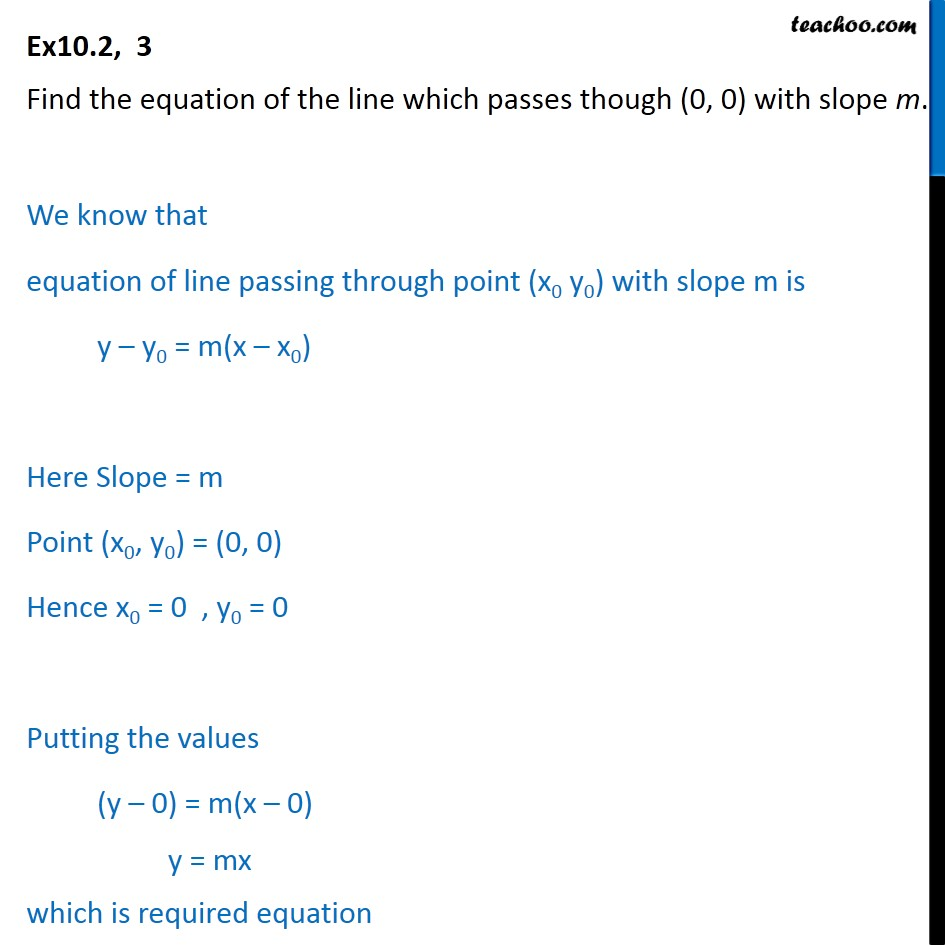Ex 9.2

Chapter 9 Class 11 Straight Lines
Serial order wiseLearn in your speed, with individual attention - Teachoo Maths 1-on-1 Class

### Transcript

Ex10.2, 3 Find the equation of the line which passes though (0, 0) with slope m. We know that equation of line passing through point (x0 y0) with slope m is y – y0 = m(x – x0) Here Slope = m Point (x0, y0) = (0, 0) Hence x0 = 0 , y0 = 0 Putting the values (y – 0) = m(x – 0) y = mx which is required equation